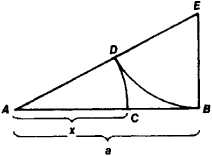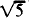# Golden Section

Also found in: Dictionary, Thesaurus, Idioms, Wikipedia.

## Golden Section,

in mathematics, division of a line segment into two segments such that the ratio of the original segment to the larger division is equal to the ratio of the larger division to the smaller division. If c is the original segment, b is the larger division, and a is the smaller division, then c = a + b and c/b = b/a. Thus, b is the geometric mean of a and c; the ratio is known as the Divine Proportion. The Golden Rectangle, whose length and width are the segments of a line divided according to the Golden Section, occupies an important position in painting, sculpture, and architecture, because its proportions have long been considered the most attractive to the eye. The constructions of regular polygons of 5, 10, and 15 sides depend on the division of a line by the Golden Section. The numerical ratio of the greater segment of the line to the shorter segment as determined by the Golden Section is symbolized by the Greek letter phi and has the approximate value 1.618. It occurs in many widely varying areas of mathematics. For example, in the Fibonacci sequence (the sequence of numbers formed by adding successive members to find the next member—0, 1, 1, 2, 3, 5, 8, 13, … ), the values of the ratios 1, 2/1, 3/2, 5/3, 8/5, 13/8, … approach the value of the Golden Section.

### Bibliography

See H. E. Huntley, The Divine Proportion (1970).

## Golden Section

the division of a line segment AB into two parts in such a way that its larger part AC is the mean proportional between the entire segment AB and its smaller part CB (see Figure 1).Figure 1

The algebraic determination of the golden section of the segment AB = a reduces to solving the equation a/x = x/(ax) (where x = AC), from which x = a (− 1)/2 ≈ 0.62a. The ratio of x to a may also be expressed approximately by the fractions ⅔, ⅗, ⅝, 8/13, 13/21, and so on, where 2, 3, 5, 8, 13, 21, and so forth are Fibonacci numbers. The geometric construction of the golden section of the segment AB is accomplished in the following manner: a perpendicular to AB is drawn at point B, the segment BE = 1/2AB is measured off, A and E are connected, ED = EB is measured off, and finally, AC = AD, thus AB/AC = AC/CB. The golden section was already known in antiquity. In the classical literature that has come down to us, the golden section is first encountered in Euclid’s Elements (third century B.C.). The term “golden section” was introduced by Leonardo da Vinci (the turn of the 16th century). The principles of the golden section and the proportional relationships close to it have served as the basis for the compositional construction of many works of world art (chiefly architectural works of ancient Greece and Rome and the Renaissance).

## golden section

[′gōl·dən ′sek·shən]
(mathematics)
The division of a line so that the ratio of the whole line to the larger interval equals the ratio of the larger interval to the smaller. Also known as divine proportion; extreme and mean ratio; golden mean; golden ratio.

## golden section

The division of a line into two segments so that the ratio of the whole line to the larger segment equals the ratio of larger segment to the smaller one; often called the golden section. This ratio was once considered by some as having an inherent aesthetic value.
References in periodicals archive ?
The golden section method does not require gradient information.
mws for implementing the Golden Section minimization and integration procedures without the various extraneous plots in SAK.
Short of boning up on your math skills, however, you can see and apply examples of the Golden Section by touring neighborhoods built prior to the Depression.
The second system is the proportional division of the work into two parts according to the golden section.
The golden section is a well-known proportion that occurs when something (e.
Recent archeological excavations have established the footprint of the first communal structure of the community; at 30 feet by 84 feet it is a double Golden Section in plan, adding credence to the possibility that the members of Ephrata lived surrounded by a mystical representation of their God, in a genuine reification in architecture of their faith.
The numerical values inherent in the sonnet structure--not only 11 and 14, but also 154 (the total number of syllables in a sonnet), 22 and 7 (the numerical elements of [pi] in their respective proportional relationships with 11 and 14), and the ratio 4:3 (or 8:6, octave to sestet)--are thus neither arbitrarily devised nor adapted from existing models in a tradition of literary forms, but chosen precisely for their power to signify key mathematical ideas, especially those connected with squaring the circle and the so-called Golden Section (in the late medieval discussion of which these very numbers, as Potters exhaustively demonstrates, recur over and over again).
The golden section can be used in floral design, especially in determining how high the flowers should be in comparison to the container (see Figure 4-15).
The Golden Rectangle has its sides in the ratio of the Golden Section.
Norgard went even further, making refined use of the golden section in rhythmic levels and limited use of the natural overtone series in harmony.
complexities which only patient and learned analysis can lay bare; and his patience even extends to examining all the masques to see how they divide at the root square and golden section.
Two gigantic but hidden pyramids - irresistibly reproduced here on fold-out sheets - turn up in the speeches of The Masque of Queens, and the climactic moment of The Golden Age Restored is found to coincide, appropriately enough, with the ratio of the Golden Section.

Site: Follow: Share:
Open / Close